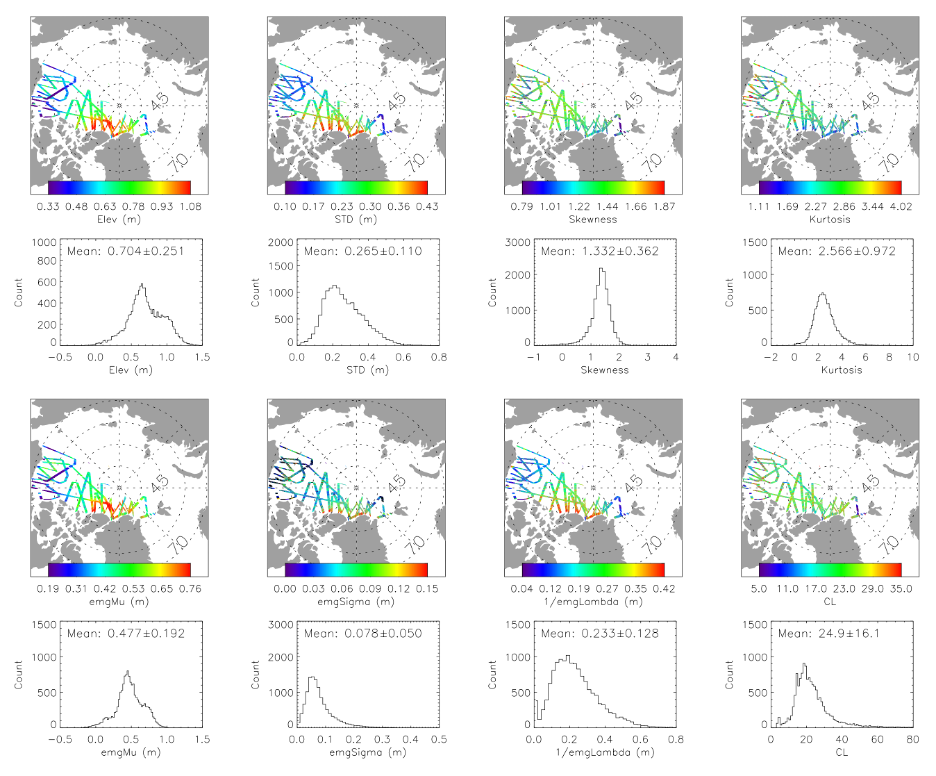# Laboratory for Satellite Altimetry / Sea Ice and Polar Dynamics Science Team

## Sea Ice Surface Elevation Distribution

The sea ice surface elevation distribution product is derived using Airborne Topographic Mapper (ATM) L1B data collected over the Arctic Ocean during NASA's Operation IceBridge (OIB) Spring campaigns between 2009 and 2019. An exponentially modified Gaussian (EMG) distribution is used to fit the surface elevation probability density function (PDF). The characteristic function of the EMG distribution can be integrated in the modeling of radar altimeter waveforms.

To build the elevation distribution, the ATM data are grouped into segments spanning 1.5 km in the along-track direction of the flight-line. Sea ice surface elevation PDF is calculated for each 1.5 km segment. Cluster analysis is used to distinguish floe heights from leads heights. Floe heights are then used for PDF calculations. The calculated PDF is then fitted to an EMG distribution model.

The EMG distribution with mean = μe + 1/λ and variance = σe2 + 1/λ2 is defined as
EMG(x)=(λ/2) e((λ/2)(2μe + λσe2-2x)) erfc⁡((μe + λσe2-x)/(√2 σe )).
where erfc is the complementary error function defined as erfc(x) = 1-erf(x) = (2/√π) ∫xe(-t2) dt.### Data Product and Data Access

Eight parameters are included in the sea ice surface elevation distribution product (e.g., Figure 1). These include four statistical parameters calculated from surface elevation measurements (mean, STD, skewness, kurtosis), three EMG model parameters from fitting to the elevation PDF (μe, 1/λ, σe), and the correlation length (CL) determined from autocovariance function calculations.

Product data files are in NetCDF format. Files are organized such that each elevation distribution product data file corresponds to an OIB ATM data file. The parameters included in an individual record include:

Data file variables

A single record of a 1.5 km segment within a netDCF file includes the variables:

```		mlat 	– mean latitude [degrees]
mlon 	– mean longitude [degrees]
melev 	– mean elevation [meters]
std 	– standard deviation of elevation [meters]
skewness 	– skewness
kurtosis 	– kurtosis
count 		– number of ATM footprints
pdf_xx 	– x-axis of elevation PDF
pdf 		– elevation pdf [counts]
Pdf_exGaussian - emg fitting of elevation pdf [counts]
exGaussian_mu - emg fitting parameter mu [meters]
exGaussian_sigma - emg fitting parameter sigma [meters]
exGaussian_lamda - emg fitting parameter lamda [meters]
exGaussian_amplitude - emg fitting parameter amplitude [counts]
ACF_xx 	- x-axis of autocorrelation function [meters]
ACF 		- Autocorrelation function [meters^2]
CL 		– correlation length [meters]